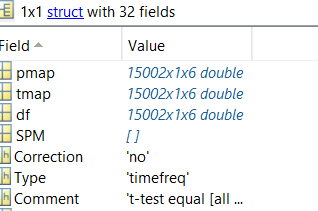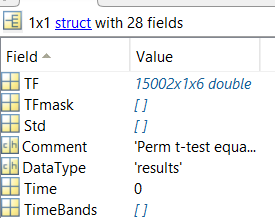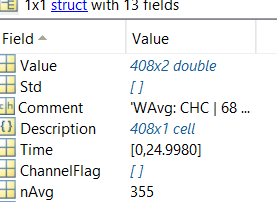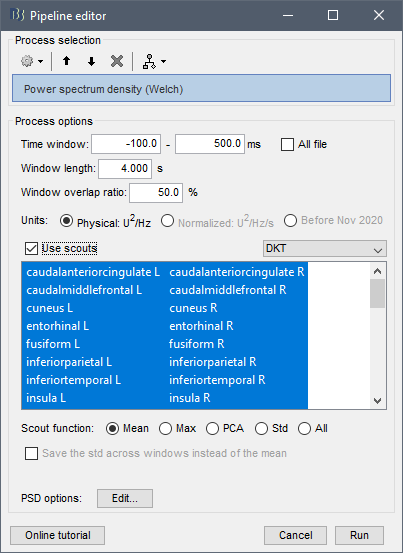1. Extraction of p-values after source statistics 2. To extract source PSD values into matlab

Hi,
I have couple of problems that i am facing

1. After we calculate source statistics for PSD or Relative power, how do we extract p-values for each ROI ( i have used Desikan-Killiany), when i do import we get 1500216 matrix. but we would want for 68 areas. How do we do that?
Same happens with permutation test as well?2. If i calculate PSD or RP values at source space then average them later to extract scout series , as table format we get 68*1 table but the same when exported to MATLAb we get 408 x 2, why is that? how do we get 68 x 1 in MATLAB?
Any matrix function similar to bst_memory('GetConnectMatrix',~) for PSD values restructuring in matlab?You can't.
If you want p-values for each ROI, you need to run your tests on the ROI time series.
Use the process Extract > Scouts time series to get one signal per ROI instead of one signal per source.

If i calculate PSD or RP values at source space then average them later to extract scout series , as table format we get 68*1 table but the same when exported to MATLAb we get 408 x 2, why is that?

I'm sorry, I don't understand what you did here.
Please explain precisely, step-by-step, what you do in order to obtain this structure.

how do we get 68 x 1 in MATLAB?

Use the option "Use scouts" in the PSD process.
Or do this is two steps: compute the ROI time series (Extract > Scout time series), and then compute the PSD from these.1 Like